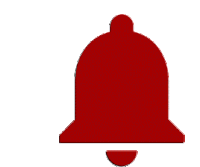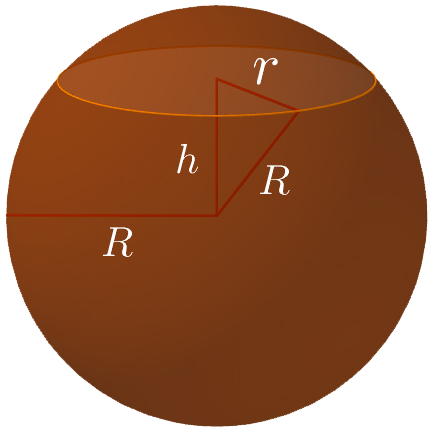# SphereArea

$A=4\pi {R}^{2}$

Volume

$V=\frac{4\pi {R}^{3}}{3}$

Surface circle

${R}^{2}={r}^{2}+{h}^{2}$
Keywords: sphere, area, volume